# Multi-pole potential

Jump to: navigation, search

potential of a multi-pole

A harmonic function on the domainin,, which is a partial derivative of some order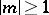of the main fundamental solution of the Laplace equation; that is, a function of the form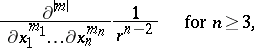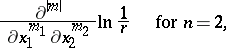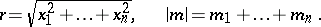For brevity, let. Fordipole potentials have the form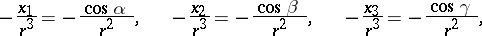where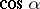,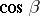and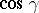are the direction cosines of the radius vector of the point of observation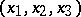. The function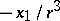, for example, is interpreted as a dipole potential with moment 1 and axis, that is, the limit as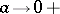of the sum of the Newton potentials of a mass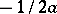placed at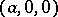, and a mass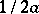placed at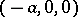; otherwise this function can be represented as the magnetic potential of a small magnet placed at the origin along the axis. Similarly, the functions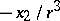and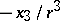are dipole potentials with axesand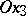, respectively. By taking linear combinations of these functions it is possible to obtain the potential of an arbitrarily oriented dipole with any moment. Fora quadrupole potential arises, obtained by a limit transition from a fixed system of four point masses whose total mass is always equal to zero, etc.

The Newton potential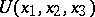of a bounded bodyof density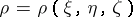, situated so that, can be expanded in a series of multi-pole potentials: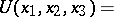(1)where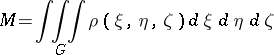is the total mass of the body, and the coefficients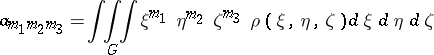are called dipole moments for, quadrupole moments for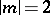, and, in general, multi-pole moments for. The series (1) differs from the expansion ofin spherical functions,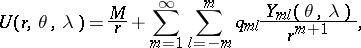(2)

by rearrangement of the terms; the terms of (2) can also be interpreted as potentials of multi-poles that are oriented in a special way (see ). Therefore the coefficients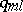are also often called, respectively, dipole, quadrupole and, more generally, multi-pole moments.

Expansions of the type (1) and (2) are used in the description and approximate representation of scalar and vector potentials, not only in connection with the fundamental solution of the Laplace equation, but also of the Helmholtz equation (see ).

In the hydrodynamics of planar flows of an ideal incompressible fluid there are also applications of complex multi-pole potentials of the formwhereis a complex variable andandare, respectively, the moment and angle of orientation of the multi-pole. The dipole potential obtained for,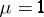andcan be interpreted as the limit asof the sum of the complex potentials of a source of capacity 1 atand a sink of capacity 1 at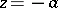. The expansion (1) here corresponds to the expansion of the complex potential of the velocity of the flow of a streamlined planar body, in a neighbourhood of the point at infinity: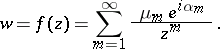Here the action of the streamlined bodyis replaced by the resultant action of multi-pole potentials placed at the origin (see ).